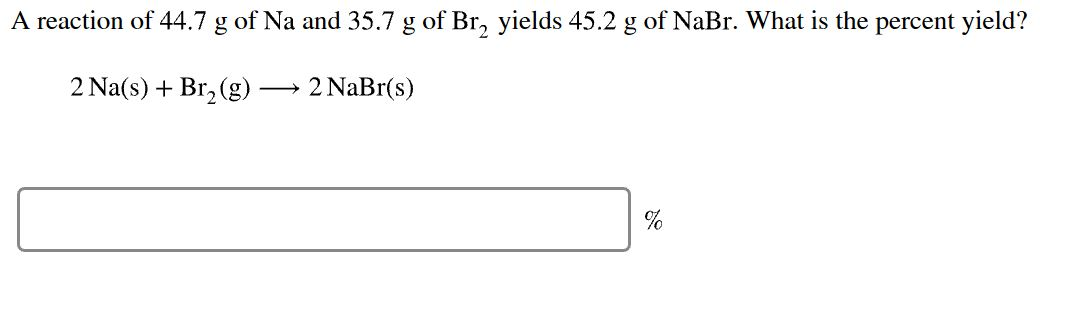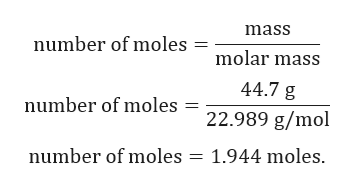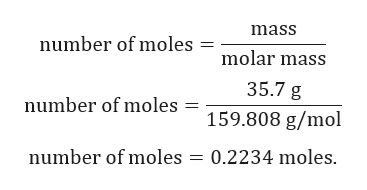# A reaction of 44.7 g of Na and 35.7 g of Br yields 45.2 g of NaBr. What is the percent yield?2 Na(s)Br2(g)2 NaBr(s)

Question
1 viewshelp_outlineImage TranscriptioncloseA reaction of 44.7 g of Na and 35.7 g of Br yields 45.2 g of NaBr. What is the percent yield? 2 Na(s)Br2(g) 2 NaBr(s) fullscreen
check_circle

Step 1

Given:

Mass of Na = 44.7 g.

Mass of Br2 = 35.7 g.

Actual yield of NaBr = 45.2 g

Balanced chemical reaction is given by:

Step 2

Calculation for moles of Na:help_outlineImage Transcriptionclosemass number of moles molar mass 44.7 g number of moles = 22.989 g/mol number of moles = 1.944 moles. fullscreen
Step 3

Calculation for moles of...help_outlineImage Transcriptionclosemass number of moles molar mass 35.7 g number of moles = 159.808 g/mol number of moles 0.2234 moles fullscreen

### Want to see the full answer?

See Solution

#### Want to see this answer and more?

Solutions are written by subject experts who are available 24/7. Questions are typically answered within 1 hour.*

See Solution
*Response times may vary by subject and question.
Tagged in

### Chemistry